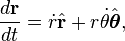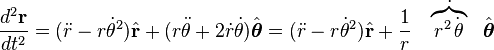# Polar coordinates

putongren
Dear All,

How do you derive both equations below. Let r be the position vector (rcos(θ), rsin(θ)), with r and θ depending on time t.

These equations can be found in wiki under polar coordinates.## Answers and Replies

Gold Member
This looks just like a staight-forward application of the chain and product rules . . .

Proof: $$\mathbf{r} = [rcos(\theta), rsin(\theta)]$$

$$\mathbf{\hat{r}} = [cos(\theta),sin(\theta)]$$

$$\mathbf{\hat{\theta}} = [-sin(\theta),cos(\theta)]$$

$$\mathbf{r} = rcos(\theta)\mathbf{e_1} + rsin(\theta)\mathbf{e_2}$$

$$\frac{d\mathbf{r}}{dt} = [\dot{r}cos(\theta) - rsin(\theta)\dot{\theta}]\mathbf{e_1} + [\dot{r}sin(\theta) + rcos(\theta)\dot{\theta}]\mathbf{e_2}$$

Expressing this in terms of our previously defined unit vectors we have that.

$$\frac{d\mathbf{r}}{dt} = \dot{r}\mathbf{\hat{r}} + r\dot{\theta}\mathbf{\hat{\theta}}$$

As desired. A similar method could probably be used to get the second result. This is probably a bit sloppy but I'm just learning vector calculus.

putongren
I'm new to vector calculus too. What does e1 and e2 mean?

Gold Member
They're unit vectors.

Tac-Tics
I'm new to vector calculus too. What does e1 and e2 mean?

The notation $$e_i$$ is often used for the i-th vector in the standard basis. So $$e_1$$ is the vector that points in the positive x direction, and $$e_2$$ to the positive y direction, etc.

putongren
Here's a good link I found on deriving those equations: http://mathworld.wolfram.com/PolarCoordinates.html" [Broken]

Last edited by a moderator:
Gold Member
Which is pretty much exactly what I showed!